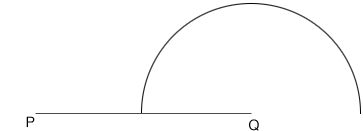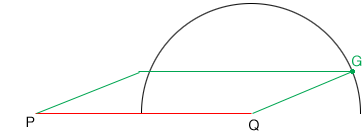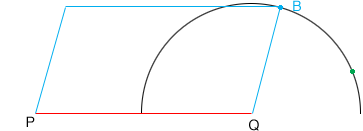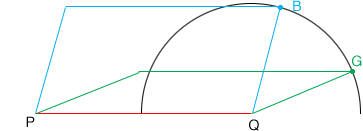SEARCH HOMEMath Central Quandaries & QueriesQuestion from ginger: Find the diagonals of parallelogram where the the side is 40 and the bottom side is 15.Hi Ginger,

There is not enough information in what you sent us to determine the lengths of the diagonals. I am going to illustrate this with some diagrams.

Draw a line $PQ$ of length 40 cm and then draw a semicircle with center $Q$ and length 15 cm. (My diagram is not to scale.)Choose a point $G$ on the semicircle, draw the line segment $QG$ and then add two line segments to complete a parallelogram. This parallelogram has sides of length 40 cm and 15 cm.Now choose a different point $B$ on the semicircle, draw the line segment $QB$ and then add two line segments to complete a parallelogram. This parallelogram also has sides of length 40 cm and 15 cm.Finally look at the two parallelograms together.Clearly the diagonals $PG$ and $PB$ are of different lengths.

PennyMath Central is supported by the University of Regina and The Pacific Institute for the Mathematical Sciences.